Editor Ratings:
User Ratings:
[Total: 0 Average: 0]

Here is a list of 10 websites to calculate Transpose of a Matrix.

These websites make it very easy and fast to calculate the transpose of any matrix. They offer different features like choosing the order of the matrix and performing different operations on matrices. Let’s see what each of the website has to offer to you.

## 1. Bluebit: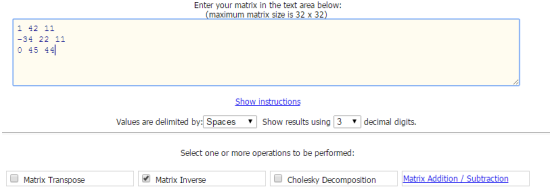Bluebit is the transpose calculator for Matrix which lets you calculate transpose in a few clicks. On opening the website, you will see the box in which you have to enter the matrix. For entering the values, you have to enter values and separate them from each other using comma, space, or tabs. After this, you need to tick the Matrix Transpose option and click on Calculate option.

Other than just calculating transpose, you can also calculate: Determinant, Matrix Rank, Matrix Inverse, Decomposition, etc..

## 2. Math Portal: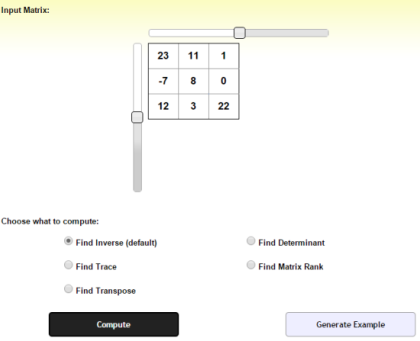Math Portal is the second transpose calculator for Matrices. To use this calculator, you first have to choose the order of the matrix and enter the corresponding values. The website includes cells in which you can enter the individual values. When you have entered the values, click on Compute button to calculate.

The Math Portal also lets you calculate Matrix Rank, Determinant, Inverse, and Trace.

## 3. Number Empire:Number Empire is an easy to use transpose calculator for any matrix. On opening this calculator, you simply have to type in the values separated by commas. When you have entered the values, tick mark the option Transpose Matrix to get the answer instantly.

There are a few more operations which you can perform like Triangular Matrix, square of matrix, inverse, determinant, etc..

## 4. Matrix Calculator: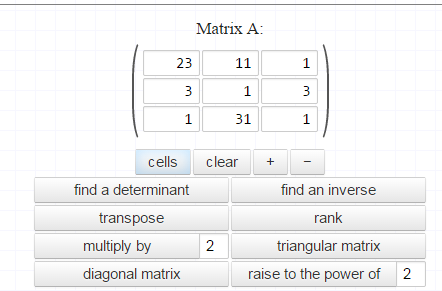The Matrix Calculator is also easy one to use with individual cells for you to enter the numbers. You can set the order of the matrix as per your choice, before you enter the problem. When you have added the values, simply click on Transpose to get the solution.

The other supported Matrix operations are: Determinant, Rank, Inverse, Multiply By, Triangular Matrix etc..

Get Matrix Calculator here.

## 5. Solve My Math: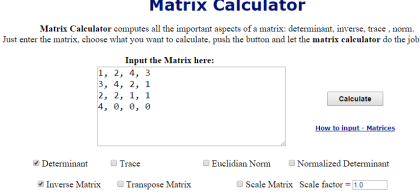The fifth transpose calculator in the list is Solve My Math. It doesn’t have preset individual cells for entering the values for solving the problem. You have to enter the values and use comma as the separator to differentiate between two different values.

Other than this, you can also calculate scale matrix, Euclidian norm, normalized determinant, determinant, etc..

## 6. Matrix Reshish: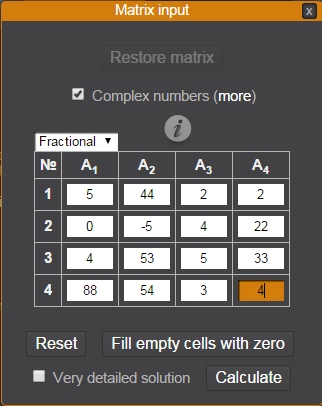The next one in the list is Matrix Reshish. It has a two-step process for calculating the transpose. On opening the web page, your first step is to choose the order of the matrix. When you have set the matrix order, you will see the pop-up to enter the values. Enter the values and click on Calculate to instantly get the transpose of the matrix entered by you.

## 7. OnlineMSchool: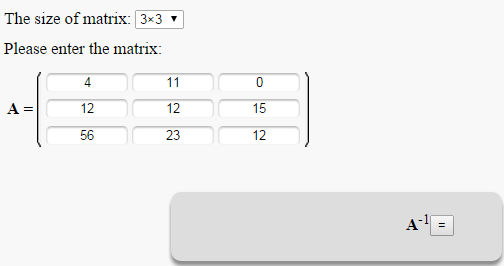The OnlineMSchool transpose calculator is the seventh one on the list. It has two separate drop down menus for selecting the number of rows and columns respectively. Depending upon the value selected by you, the matrix size gets adjusted automatically. Since it has individual cells for entering values so you don’t have to use separators. Simply enter the value and click on Transpose button to get the result.

## 8. TutorVista: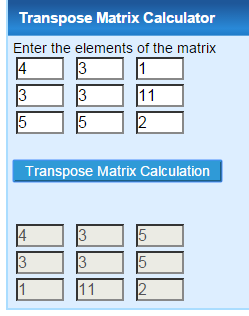TutorVista has one of the easiest to use transpose calculator in this list. On opening the calculator, you will see two matrices. The matrix at the top is for entering the problem you want to solve. When you have entered the value, click on Transpose Matrix Calculation button to get the output.

The calculator displays the result in the matrix below the input matrix.

Update 2022: TutorVista doesn’t exist anymore. Use Matrix Transpose Calculator of AztoZmath instead.

## 9. nCalculators: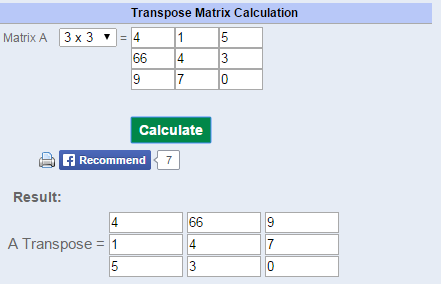Next in our list of transpose calculators is nCalculators. Its interface is somewhat similar to the TutorVista. You have to enter the matrix at top and you get the answer in the matrix at bottom. You can select the order of the matrix using the drop down menu at left. The other good feature of this calculator is that it also lets you print the result.

## 10. Easy Calculation:The last transpose calculator in the list is Easy Calculation. It lets you enter values by using comma as the separator. There are two options: Calculate and Reset. Click on Calculate to get the solution and click on Reset to reset the values entered by you.

These were the 10 websites using which you can calculate transpose of a matrix easily. Do give them a try.

You may also like: 10 websites to calculate Inverse and Determinant of a Matrix.

 Editor Ratings: User Ratings:[Total: 0 Average: 0] Free/Paid: Free Tags: Matrix calculator